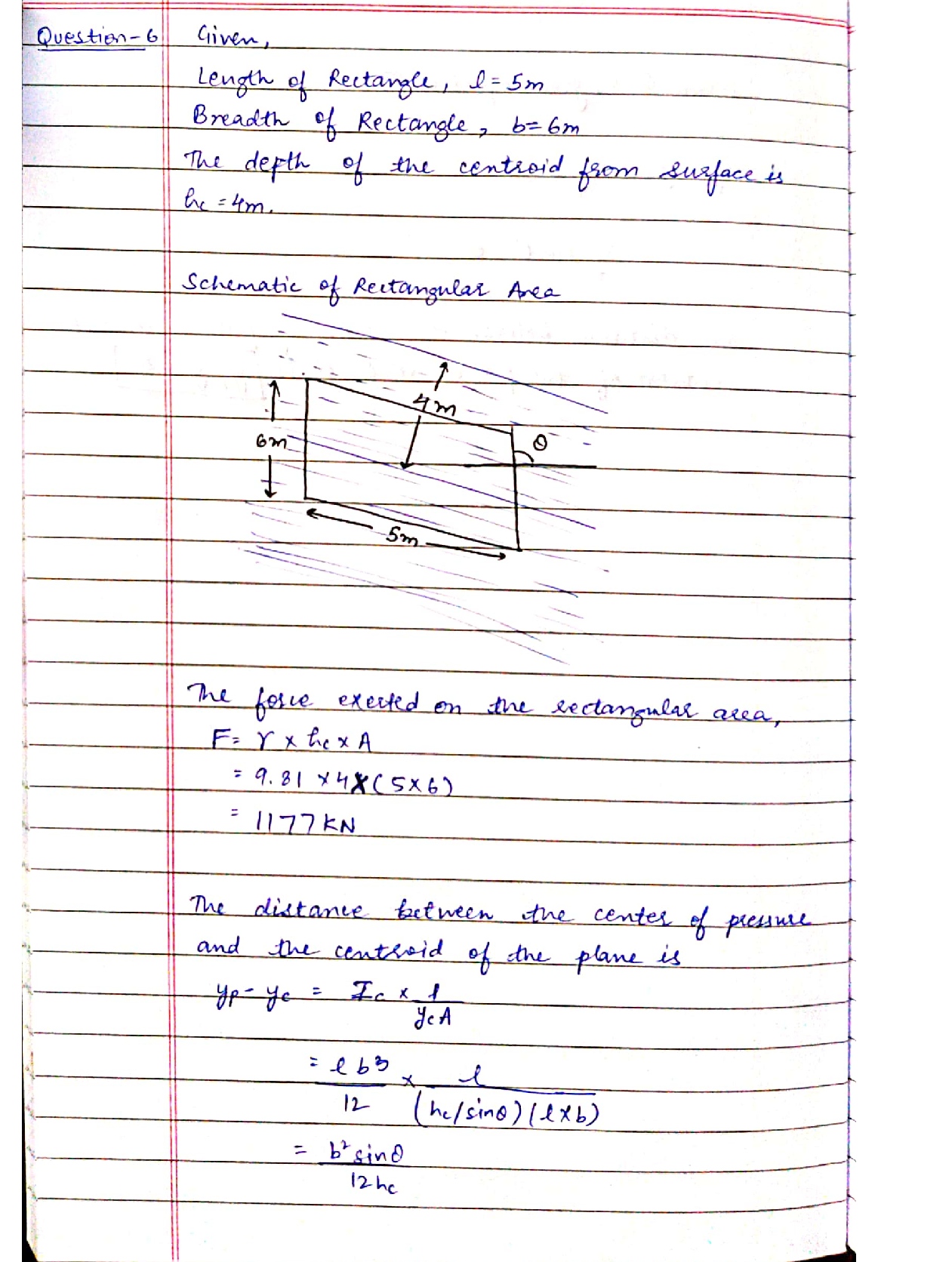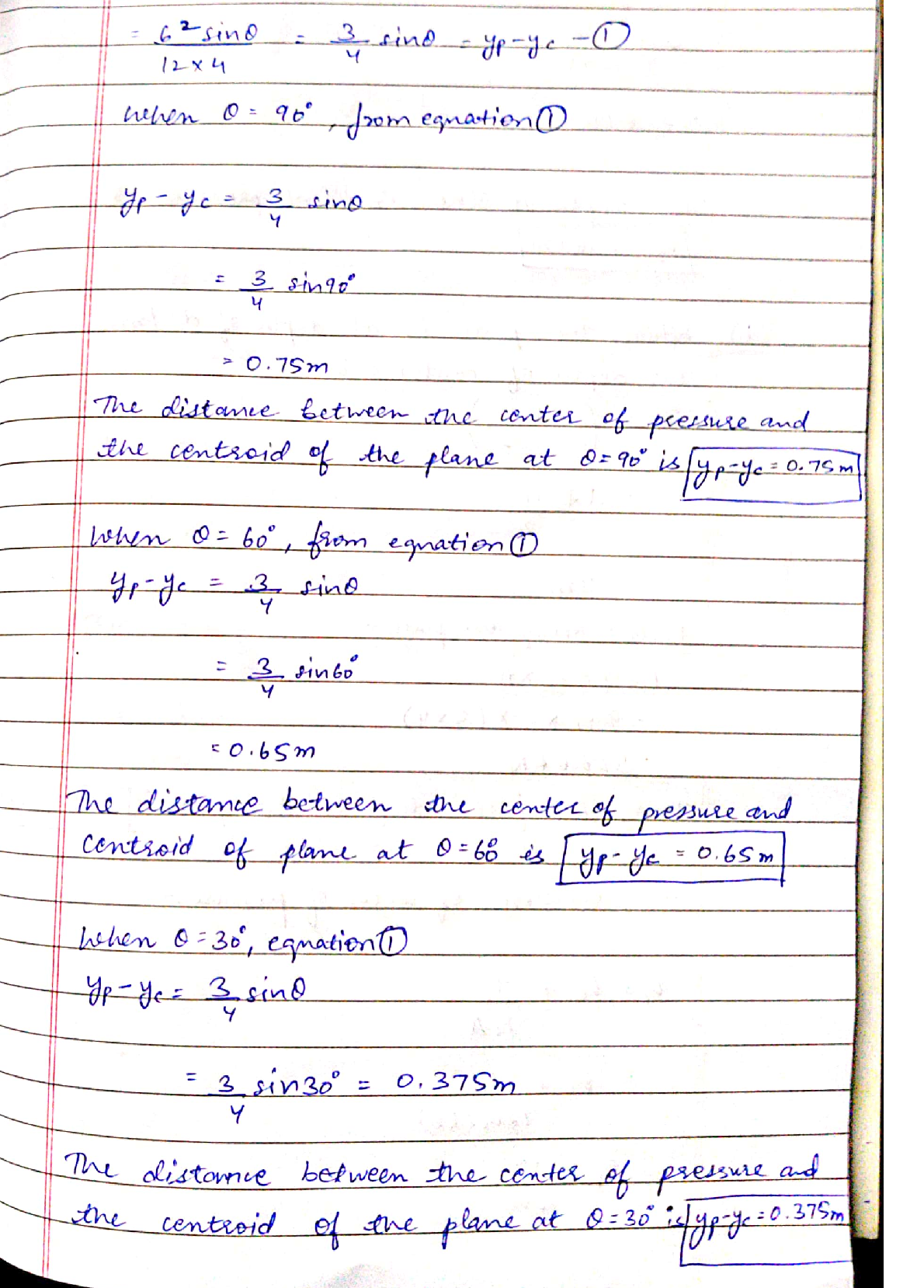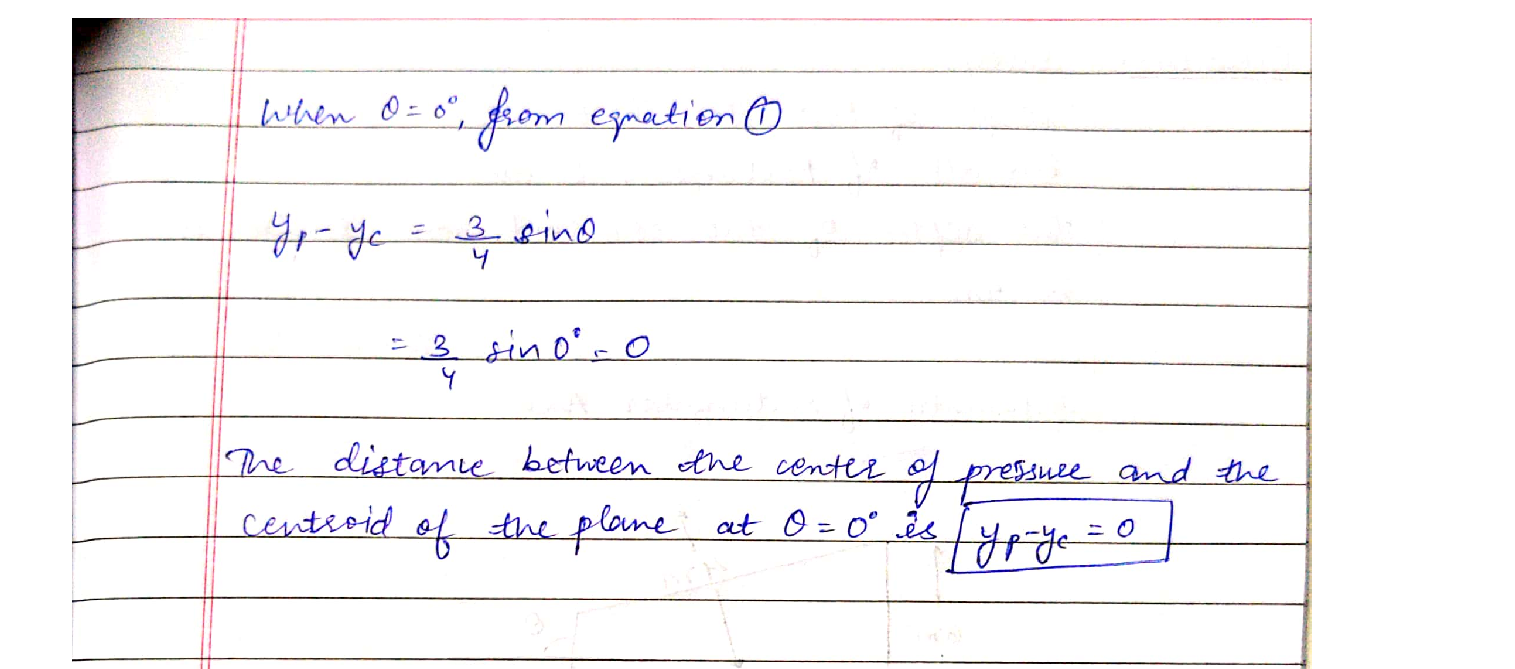Question

Intoduction To Earth Sciences

Problem-6. A rectangular area is 5 m by 6 m, with the 5 m side horizontal. It is placed with its centroid 4m below a water surface and rotated about horizontal axis in the plane area and through its centroid.Find the magnitude of the force on one side and the distance between the center of pressure and the centroid of the plane when the angle with horizontal, 0 = 90°, 60°, 30°, and 0°.Verified### Question 25135Intoduction To Earth Sciences

Problem-8. Repeat Problem-7 where the tank is open at the top and contains water to a depth of h = 10ft.

### Question 25134Intoduction To Earth Sciences

Problem-7. The hemispherical body shown in the Figure (r 2 ft) projects into a tank. Find the horizontal and vertical forces acting on the hemispherical projections for the following cases: (a) the tank is full of water with the free surface 5 ft above A;(b) the tank contains CCI4 (s = 1.59) to the level of A overlain with water having its free surface 5 ft above A; (c) the tank is closed and contains only gas at a pressure of6 psi; (d) the tank is closed and contains water to the level of A overlain with gas at a pressure of 2 psi. Assume the gas weighs 0.075 lb/ft3.

### Question 25133Intoduction To Earth Sciences

Problem-6. A rectangular area is 5 m by 6 m, with the 5 m side horizontal. It is placed with its centroid 4m below a water surface and rotated about horizontal axis in the plane area and through its centroid.Find the magnitude of the force on one side and the distance between the center of pressure and the centroid of the plane when the angle with horizontal, 0 = 90°, 60°, 30°, and 0°.

### Question 25132Intoduction To Earth Sciences

Problem-5. A rectangular plate submerged in water is 5 m by 4 m, the 5-m side being horizontal and the4-m side being vertical. Determine the magnitude of the force on one side of the plate and the depth to its center of pressure if the top edge is (a) at the water surface; (b) 1.0 m below the water surface; (c)100 m below the water surface.

### Question 25131Intoduction To Earth Sciences

Problem-4. The gate MN in the Figure rotates about an axis through N. If a= 3.3 ft, b = 1.3 ft, d = 2 ft, and the width perpendicular to the plane of the figure is 3.0 ft, what torque applied to the shaft through N is required to hold the gate closed?

### Question 25130Intoduction To Earth Sciences

Problem-3. A rectangular plate 5 ft by 4 ft is at an angle of 30° with the horizontal, and the 5-ft side is horizontal. Find the magnitude of the force on one side of the plate and the depth of its center of pressure when the top edge is (a) at the water surface, (b) 1.0 ft below the water surface.

### Question 25129Intoduction To Earth Sciences

Problem-2. Find the magnitude and depth of the point of application of the force on the circular gate shown in the Figure, if h 5 ft and D = 4 ft diameter.

### Question 25128Intoduction To Earth Sciences

Problem-1. A plane surface is circular with a diameter of 2 m. If it is vertical and the top edge is 0.5 m below the water surface, find the magnitude of the force on one side and the depth to the center of pressure.

### Question 17860Intoduction To Earth Sciences

1.Your description should include:Provide a description of the physiographic and geologic setting of your hometown.
(10 pts) The city, county, and state as well as the Latitude and Longitude of the location that you considered.
) (15 pts) The physiographic section and district of your location and a brief description of this section/district
(15 pts) The geologic formation(s) that are present at your location and the published description of the formation(s).
(10 pts) Supporting maps showing the physiography and geology of the area with your location marked on them.
Show you some online tools for accessing geologic and physiographic data and maps
introduce general types of soil how they look feel and behave
•learn about the real world incomings of key soil properties

### Question 10828Intoduction To Earth Sciences

9. Its midnight and the moon is just rising on the Eastern horizon. What phase is it in?

### Submit query

Getting answers to your urgent problems is simple. Submit your query in the given box and get answers Instantly.

### Submit a new QuerySuccess

Assignment is successfully created